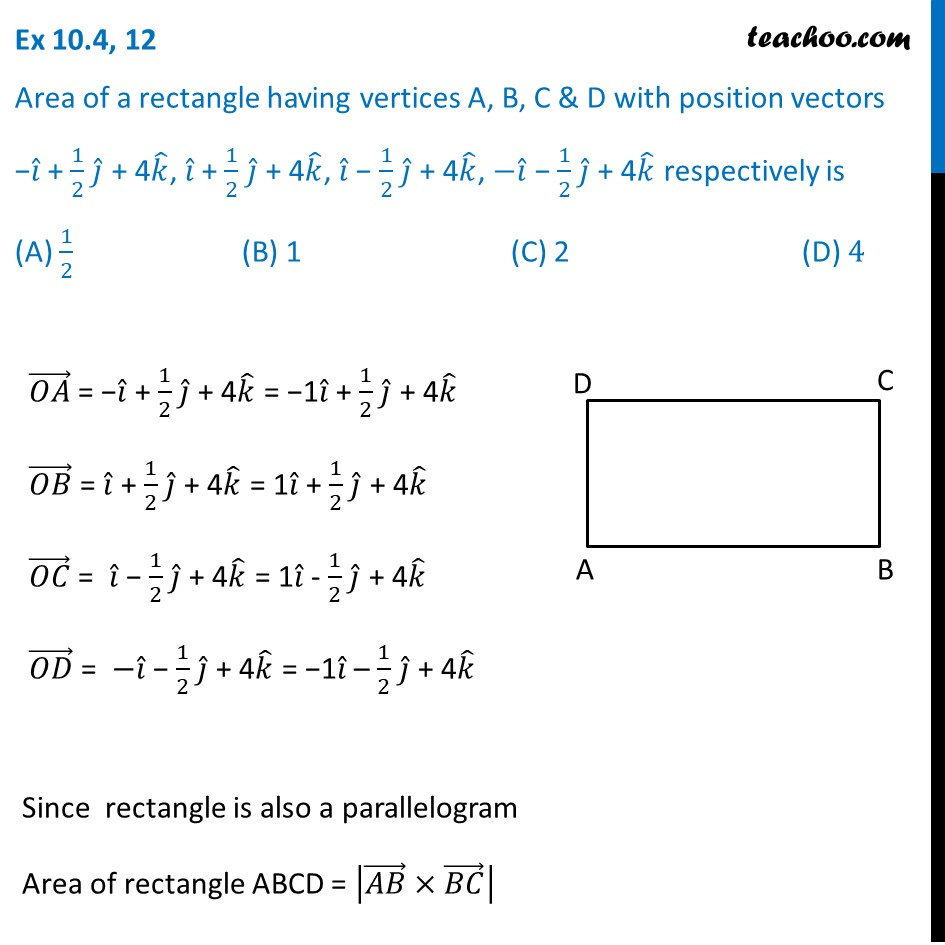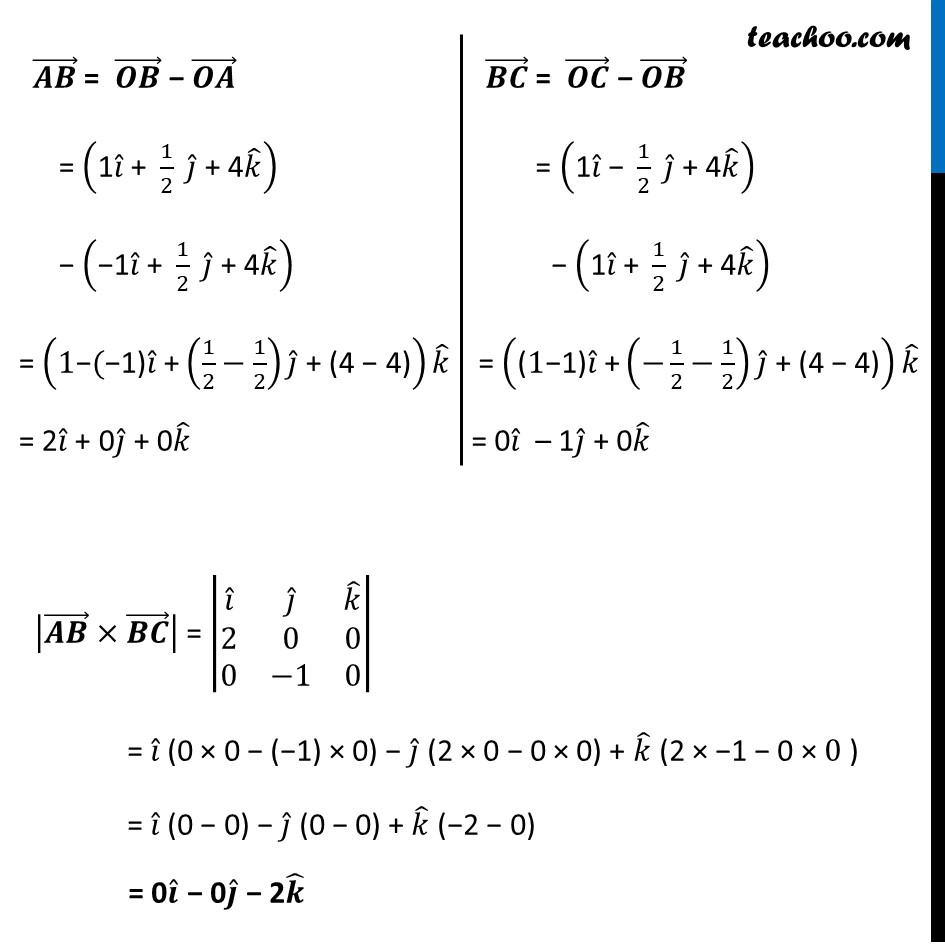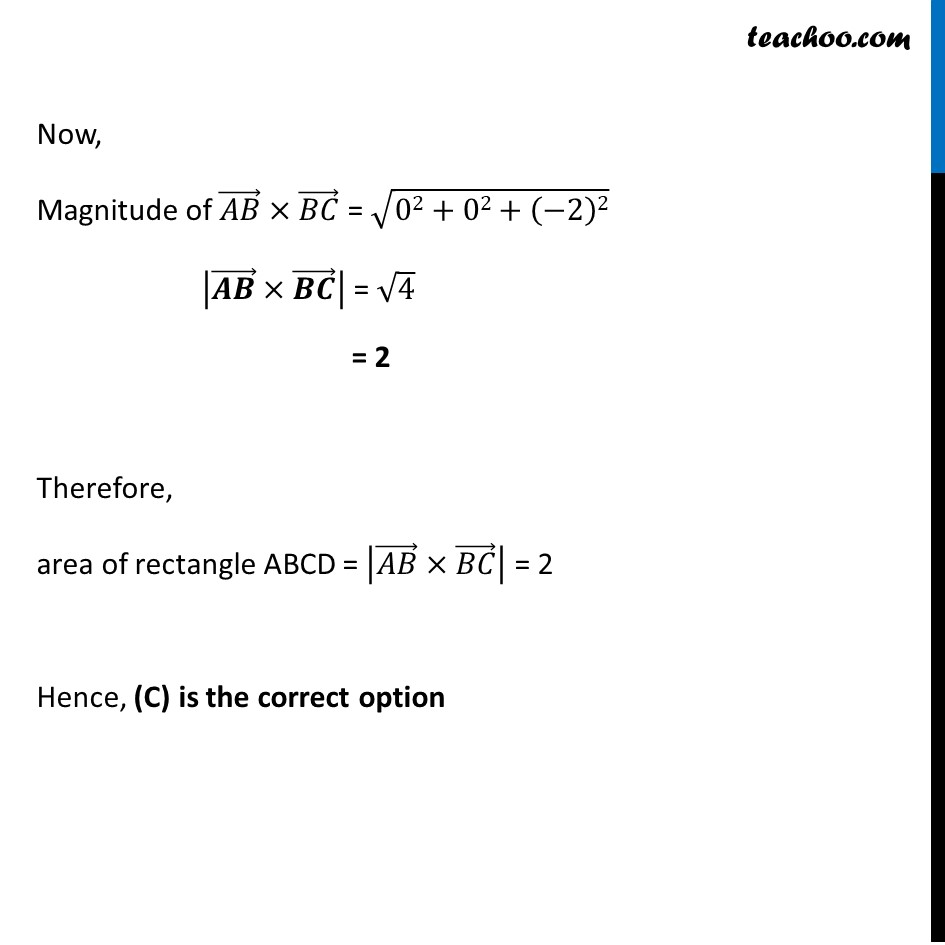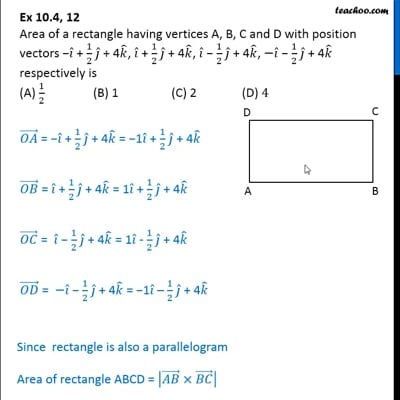Vector product - Area

Chapter 10 Class 12 Vector Algebra
Concept wiseThis video is only available for Teachoo black users

Introducing your new favourite teacher - Teachoo Black, at only ₹83 per month

### Transcript

Ex 10.4, 12 Area of a rectangle having vertices A, B, C & D with position vectors −𝑖 ̂ + 1/2 𝑗 ̂ + 4𝑘 ̂, 𝑖 ̂ + 1/2 𝑗 ̂ + 4𝑘 ̂, 𝑖 ̂ − 1/2 𝑗 ̂ + 4𝑘 ̂, −𝑖 ̂ − 1/2 𝑗 ̂ + 4𝑘 ̂ respectively is (A) 1/2 (B) 1 (C) 2 (D) 4 (𝑂𝐴) ⃗ = −𝑖 ̂ + 1/2 𝑗 ̂ + 4𝑘 ̂ = −1𝑖 ̂ + 1/2 𝑗 ̂ + 4𝑘 ̂ (𝑂𝐵) ⃗ = 𝑖 ̂ + 1/2 𝑗 ̂ + 4𝑘 ̂ = 1𝑖 ̂ + 1/2 𝑗 ̂ + 4𝑘 ̂ (𝑂𝐶) ⃗ = 𝑖 ̂ − 1/2 𝑗 ̂ + 4𝑘 ̂ = 1𝑖 ̂ - 1/2 𝑗 ̂ + 4𝑘 ̂ (𝑂𝐷) ⃗ = −𝑖 ̂ − 1/2 𝑗 ̂ + 4𝑘 ̂ = −1𝑖 ̂ – 1/2 𝑗 ̂ + 4𝑘 ̂ Since rectangle is also a parallelogram Area of rectangle ABCD = |(𝐴𝐵) ⃗×(𝐵𝐶) ⃗ | (𝑨𝑩) ⃗ = (𝑶𝑩) ⃗ − (𝑶𝑨) ⃗ = ("1" 𝑖 ̂" + " 1/2 " " 𝑗 ̂" + 4" 𝑘 ̂ ) − ("−1" 𝑖 ̂" + " 1/2 " " 𝑗 ̂" + 4" 𝑘 ̂ ) = ("1−(−1)" 𝑖 ̂" +" (1/2−1/2) 𝑗 ̂" + (4 − 4)" ) 𝑘 ̂ = 2𝑖 ̂ + 0𝑗 ̂ + 0𝑘 ̂ (𝑩𝑪) ⃗ = (𝑶𝑪) ⃗ − (𝑶𝑩) ⃗ = ("1" 𝑖 ̂" − " 1/2 " " 𝑗 ̂" + 4" 𝑘 ̂ ) − ("1" 𝑖 ̂" + " 1/2 " " 𝑗 ̂" + 4" 𝑘 ̂ ) = ("(1−1)" 𝑖 ̂" +" (−1/2−1/2) 𝑗 ̂" + (4 − 4)" ) 𝑘 ̂ = 0𝑖 ̂ – 1𝑗 ̂ + 0𝑘 ̂ |(𝑨𝑩) ⃗×(𝑩𝑪) ⃗ | = |■8(𝑖 ̂&𝑗 ̂&𝑘 ̂@2&0&0@0&−1&0)| = 𝑖 ̂ (0 × 0 − (−1) × 0) − 𝑗 ̂ (2 × 0 − 0 × 0) + 𝑘 ̂ (2 × −1 − 0 × 0 ) = 𝑖 ̂ (0 − 0) − 𝑗 ̂ (0 − 0) + 𝑘 ̂ (−2 − 0) = 0𝒊 ̂ − 0𝒋 ̂ − 2𝒌 ̂ Now, Magnitude of (𝐴𝐵) ⃗×(𝐵𝐶) ⃗ = √(02+02+(−2)2) |(𝑨𝑩) ⃗×(𝑩𝑪) ⃗ | = √4 = 2 Therefore, area of rectangle ABCD = |(𝐴𝐵) ⃗×(𝐵𝐶) ⃗ | = 2 Hence, (C) is the correct option